• 0

# Golden Ratio

Go to solution Solved by Natwar Lal,

Golden Ratio is an irrational number also denoted by Greek letter 'phi' with a value of 1.618. Two numbers are said to be in a golden ratio if the ratio of these numbers (larger value to smaller value) is the same as the ratio of their sum to the larger of the two numbers. It has a lot of other names as well e.g. - golden mean, golden proportion, divine proportion etc.

An application-oriented question on the topic along with responses can be seen below. The best answer was provided by Natwar Lal on 2nd Oct 2020.

Applause for all the respondents - Natwar Lal, Aritra Das Gupta, Sourabh Nandi.

## Question

Q 301. Golden Ration or Phi is an irrational number (=1.618). This rather ordinary looking number is present everywhere in nature and is extensively used by designers. What is its relevance and give examples of its applicability in business.
(One providing maximum number of correct examples will be the winner)

Note for website visitors - Two questions are asked every week on this platform. One on Tuesday and the other on Friday.

## Recommended Posts

• 0
• Solution

Let us consider two numbers a and b where a is greater than b. If the ratio of the sum of these numbers (i.e. a+b) to the larger number (i.e. a) is same as the ratio of the numbers (i.e a is to be), then these two numbers are said to be in a Golden Ratio.

Golden Ratio => (a+b) / a = a / b

This is denoted by Greek letter phi (or). This ratio comes to an irrational number = 1.618

Applicability of Golden Ratio is found in

1. Nature - sunflower, position of leaves

2. Architecture

3. Art

4. Music

5. Technical Analysis of Stocks

6. Design Thinking - laptop screens, mobile screen size

7. Book layouts and publishing

8. Logo designs - Twitter and Apple

9. Computer algorithms

10. Mathematics - fibonacci series

11. Geometry - spiral shapes

##### Share on other sites
• 0

Golden Ratio is also known as the golden mean,golden section, divine proportion.

The golden number is 1.61803399 is denoted by the greek letter phi.  When a line has 2 parts one is short and the other is long . If the long part is divided by the short part and is equal to the whole length of the line divided by the long part it is the golden ratio.The Actual Value is :

1.61803398874989484820.....

Golden Ratio is largely used in design :-

1.Website Font Size -  Golden ratio is used to define the size of  the headline or the text . This provides a symmetry to the text and gives aesthetic value to the writing as well as ensures that its easily readable.

2.Cropping of Image

3. Logo development

4. Product design uses this concept

5.Artistic composition and historic monuments also follow this .Example Taj Mahal,Pyramid also follows this.

##### Share on other sites
• 0

The Golden Ratio is a mathematical ratio found almost anywhere, like nature, architecture, painting, photography, and music. When applied to design specifically, it creates an organic, balanced, and aesthetically-pleasing composition.

The Golden Ratio is derived when we divide a line into two parts and the longer part (a) separated by, the smaller section (b) is equal to the sum of (a) + (b) divided by (a), which both equal to 1.618. This formula helps us to create shapes, logos, layouts, and more.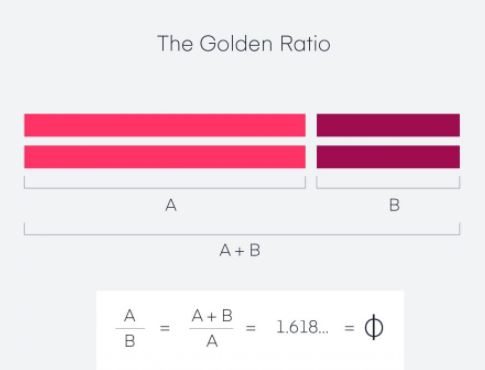Using the same idea, we can create a golden rectangle. The below diagram shows a rectangle with harmonious proportions using a square and multiple sides by 1.618 to get a new shape of a rectangle with balanced proportions.

While applying the Golden Ratio formula to the new rectangle, we end up with an image made up of increasingly smaller squares. Therefore, spiral over each square, starting in one corner and ending in the opposite one, we get the Fibonacci sequence (also known as the Golden Spiral).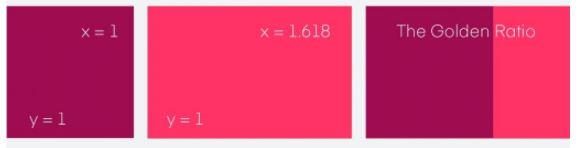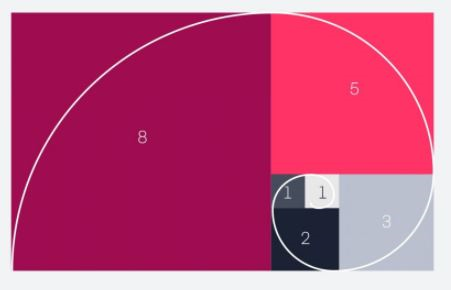Example1: Used for a Logo Design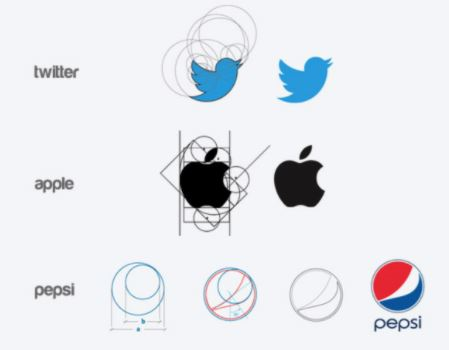Example2: Cropping and resizing images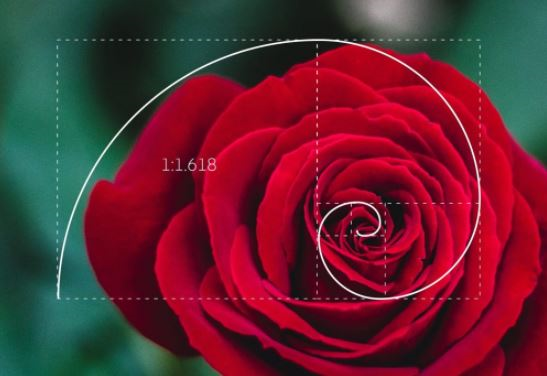Example3: Typography and defining hierarchy

The Golden Ratio helps us find font size used for headers and body copy on a website, landing page, blog post, or even print drive.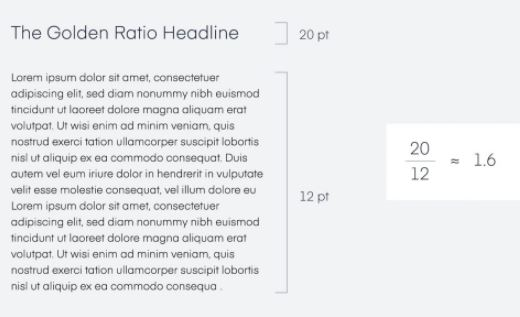##### Share on other sites
• 0

While all published answers have clearly and correctly defined Golden Ration, Natwar Lal has given the maximum number of examples for applicability in various businesses and hence has been selected as the best answer. Congratulations!

##### Share on other sitesThis topic is now closed to further replies.
• ### Who's Online (See full list)

There are no registered users currently online

• ### Forum Statistics

• Total Topics
2,876
• Total Posts
14,575
• ### Member Statistics

• Total Members
55,085
• Most Online
888